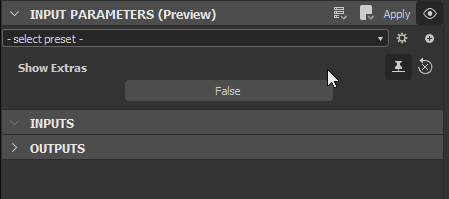User Guide Cancel

# Visible if: control visibility of inputs, outputs and parameters

This page explains the "Visible if" feature in Adobe Substance 3D Designer, which lets you control the visibility of inputs, outputs and parameters in graphs.

## Overview

When exposing parameters, you may want to hide or show parameters or node connectors based on the status of other parameters. E.g., a slider only showing when a boolean parameter button is set to `true`, because it would have no effect otherwise and that might confuse users.

To achieve this, you may input a logical expression into the Visible if property of:If the logical expression evaluates to `true`, the parameter, input or output is displayed in all instance nodes representing the current graph. Otherwise, it is hidden.

Complex conditions are possible, provided the logical expression stating these conditions is valid.

Note:

This feature only impacts whether a parameter or connector is displayed in the user interface, and has no effect on the computations and result of a graph.

Note:

This functionality works inside the Substance 3D ecosystem. Some integrations such as the Substance in Maya plugin support it as well, but not all of them. If unsupported, the visibility  condition defaults to `true`.

## Writing "visible if" expressions

### ACCESSING INPUT PARAMETERS

Any Visible If Expression will need to use at least one input, this can be done through the following syntax:

```input.identifier
input["identifier"]```

The identifier must be the exact name of an existing input parameter's Identifier property, and it must be typed case-sensitive. You cannot refer to a parameter by its Label.
If a referenced parameter does not exist, or the logical expression is invalid, a warning is displayed on the Visible if property.

### AVAILABLE OPERATORS

The "Visible if" fields accepts the following parameters:

• Boolean, Float and Integer inputs.
• `true` and `false` values (case sensitive, no caps!)
• `.x` : access the subparameter
• `&&` : and
• `||` : or
• `!` : not
• `<`, `>`, `<=`, `>=`, `==`, `!=` : comparison
• ` ()` : brackets

### MUST ALWAYS EVALUATE TO BOOLEANS

A Visible If Expression is used as the condition for an "IF" statement, that means it must always result in `true` or `false`.

• Boolean values can directly evaluate as the condition. A simple button with a boolean value requires no more than this. See below examples, first case;
• Non-boolean parameters generally require a comparison operation. See above for comparison operators, below for examples;
• Some non-boolean values can be truthy or falsy, which means they can evaluate as `true` of `false` – E.g. an integer value of `0` evaluates to false.

## Examples

Condition ("If")

Formula

Note

True

```input["my_input"]
input.my_input```
```input["my_input"] == true
input.my_input == true```

my_input is a boolean value

False

```!input["my_input"]
!input.my_input```
```input["my_input"] == false
input.my_input == false```
```input["my_input"] != true
input.my_input != true```

my_input is a boolean value

Lower than

```input["my_input"] < 3
input.my_input < 3```

my_input is an integer value

Equal

```input["param1"] == 2
input.param1 == 2```

param1 is a float or integer value

Lower than

```input["my_input"].y < 3
input.my_input.y < 3```

my_input is a float or integer value with one or more components – e.g. float2(x, y), integer3(x, y, z)

Or

```input["param1"] || input["param2"]
input.param1 || input.param2```

param1 and param2 are boolean values

And

```input["param1"] > 0 && input["param2"] > 1
input.param1 > 0 && input.param2 > 1```

param1 and param2 are float or integer values